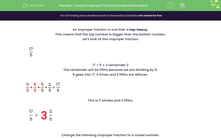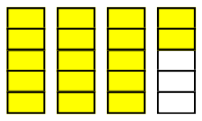# Convert Improper Fractions to Mixed Numbers

In this worksheet, students will practise converting improper fractions to mixed numbers.Key stage:  KS 2

Curriculum topic:   Number: Fractions, Decimals and Percentages

Curriculum subtopic:   Convert Fractions

Difficulty level:#### Worksheet Overview

An improper fraction is one that is top-heavy.

This means that the top number is bigger than the bottom number.

Let's look at this improper fraction:

 17 5

17 ÷ 5 = 3 remainder 2

The remainder will be fifths because we are dividing by 5.

5 goes into 17, 3 times and 2 fifths are leftover.

 5 + 5 + 5 + 2 = 17 5 5 5 5 5

This is 3 wholes and 2 fifths.

 17 = 3 2 5 5Change the following improper fraction to a mixed number:

 39 7

39 ÷ 7 = 5 remainder 4

This is 5 wholes and 4 sevenths.

 39 = 5 4 7 7

### What is EdPlace?

We're your National Curriculum aligned online education content provider helping each child succeed in English, maths and science from year 1 to GCSE. With an EdPlace account you’ll be able to track and measure progress, helping each child achieve their best. We build confidence and attainment by personalising each child’s learning at a level that suits them.

Get started